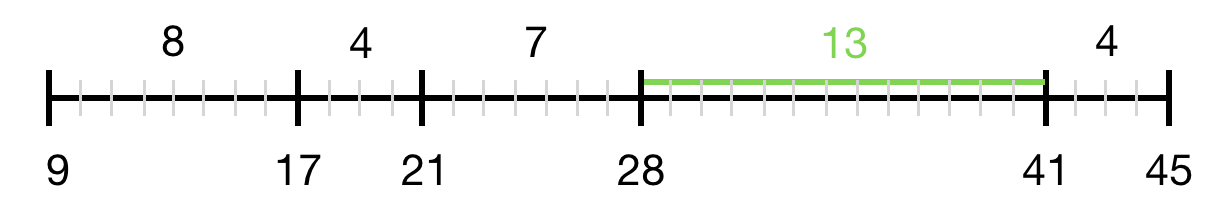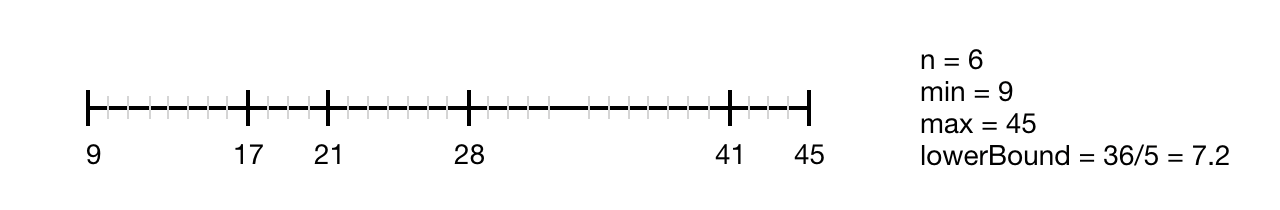Career coaching for women in tech.

# My Favorite Linear-time Sorting Algorithm

Counting sort with a twist

The problem: Given an unsorted array of numbers, find the maximum difference between the successive elements in its sorted form. The numbers can be negative or decimals.Given [21, 41, 17, 45, 9, 28], the maximum difference is 13.

## Straightforward Algorithm

``````const maxGap = input =>
input
.sort((a, b) => a — b)
.reduce((acc, cur, idx, src) =>
Math.max(acc, idx > 0 ? cur — src[idx — 1] : 0), 0);
``````

This function first sorts the inputs. Resulting in `[9, 17, 21, 28, 41, 45]` for our example. Next, it iterates over the sorted array and keeps track of the maximum difference in the accumulator variable:

• max(0, 0) = 0
• max(0, 8) = 8
• max(8, 4) = 8
• max(8, 7) = 8
• max(8, 13) = 13
• max(13, 4) = 13

The complexity for this solution is `O(n*log(n))` for sorting, and `O(n)` for finding the maximum difference, resulting in `O(n*log(n))`. `n` is the number of elements in the input. Any comparison sort has a lower bound of `O(n*log(n))`, so we cannot find a faster solution using comparison sort — but there is a linear time solution to this problem. Let’s find it!

## Linear Time Solution

Comparison sorts like mergesort, heapsort, or quicksort are too slow if we look for a linear time solution. What about counting sort? Counting sort is an integer sorting algorithm that has runtime complexity `O(n+w)` and requires additional space `w` where `w` is the maximum element in the unsorted array. It works well if the integers are small. Counting sort uses a second array of length `w`, a count or frequency array.

### Counting Sort Algorithm

• For every input `i`, increase the value at index `i` in the frequency array.

• Iterate over the frequency array. For every index where the value is greater than 0 in the frequency array, push the index to the solution array.

Filling the frequency array takes `O(n)`, iterating over the frequency array and pushing elements into a sorted array takes `O(w)`. The total runtime is `O(n+w)`.

Unfortunately, a regular counting sort is not applicable to our problem because numbers can be negative, numbers can be floating point numbers, and numbers can be large.

But there is one crucial observation that allows us to use a modified counting sort that runs in linear time and requires linear additional space. Let max and min be the largest and smallest numbers of the input, respectively. The maximum difference is bounded below by `lowerBound = (max-min)/(n-1)`.The maximum distance is exactly 7.2, if all 6 numbers are evenly spread out between 9 and 45.No matter what the four numbers between 9 and 45 are, there are always two numbers that are at least 7.2 apart.

Using this observation, we can divide the range of numbers into `(n-1)` buckets of length `lowerBound`. The maximum gap does not fit within one bucket.

We map every number `i` to its bucket. Bucket sort performs poorly if numbers are clustered because numbers in the same bucket must be sorted. We get around this problem by storing only the minimum and maximum in every bucket. We can drop the middle numbers because `lowerBound` is as large as a bucket.

We find the maximum difference by iterating over the array of buckets of length `(n-1)` where every bucket has at most two entries.

``````const maxGap = (arr) => {
const n = arr.length;
let min = Number.POSITIVE_INFINITY;
let max = Number.NEGATIVE_INFINITY;
for (let i = 0; i < n; i++) {
min = Math.min(arr[i], min);
max = Math.max(arr[i], max);
}

let range = max - min;
let lowerBound = range / (n - 1);

let buckets = []; // n-1 buckets, 2 elements each => linear space complexity
for (let i = 0; i < n; i++) {
let index = Math.floor((arr[i] - min) / lowerBound);
if (!buckets[index]) {
buckets[index] = {};
buckets[index].left = arr[i];
buckets[index].right = arr[i];
} else {
if (buckets[index].left > arr[i]) {
buckets[index].left = arr[i]
}
if (buckets[index].right < arr[i]) {
buckets[index].right = arr[i]
}
}
}

let maxDiff = 0;
let prev = min;
for (let i = 0; i < buckets.length; i++) {
if (!buckets[i]) {
continue;
}
if (buckets[i].left - prev > maxDiff) {
maxDiff = buckets[i].left - prev;
}
prev = buckets[i].right;
}

return maxDiff;
}
``````

This algorithm runs in linear time, independent of how far the input numbers are apart from each other. It requires linear additional space.

## Performance

`O(n)` is faster than `O(n*log(n))` for large enough inputs. What does that mean in practice for our solutions?

For small inputs with less than 10000 elements, the straightforward function that uses `array.prototype.sort()` is faster than the linear approach. For large inputs, the linear algorithm is faster. For example for 10000000 elements, it takes 4 seconds instead of 15 seconds.

``````Array with 1000 elements:
n log(n) takes 0 seconds
Linear takes 0.001 seconds

Array with 10000 elements:
n log(n) takes 0.012 seconds
Linear takes 0.001 seconds

Array with 5000000 elements:
n log(n) takes 5.914 seconds
Linear takes 1.793 seconds

Array with 10000000 elements:
n log(n) takes 15.611 seconds
Linear takes 3.896 seconds
``````

## Conclusion

Counting sort is an integer sorting algorithm that runs in `O(n)`. All comparison sorts take at least `O(n*log(n))`.

Since the maximum difference has a lower bound, we can use a linear time algorithm, a variation of counting sort and bucket sort.

The full code is on GitHub and there’s a video of me on YouTube live coding the solution.

Subscribe to the newsletter
I'll only send emails when new content is posted. No spam.